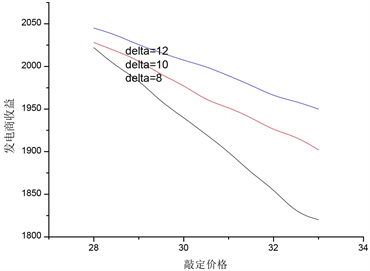﻿ 考虑期权合约的电力市场纳什均衡分析

# 考虑期权合约的电力市场纳什均衡分析Analysis of Nash Equilibrium for Electricity Markets Considering Options Contracts

Abstract: The electricity financial derivatives product market, which consists of outside-market products such as forward contracts and options contracts, provides an effective place for market participants to avoid short-period market behaviors such as price risk in the power market by using financial derivatives. However, there is little research on the impact of options on the bidding strategies of power suppliers. This paper establishes a game model of market equilibrium based on the consideration of electricity options and the number of generators in the market to improve the market efficiency and maintain the stability of market price and income. The results show that the existence of options to a certain extent inhibits the abuse of market power by generators, improves the market efficiency, and encourages more generators to join options. In the market, maintaining the high volatility of spot electricity price is conducive to maintaining stable income and high spot electricity price.

1. 引言

2. 模型的基本构想

$\lambda =r-sq+\epsilon =r-s\underset{i\in N}{\sum }{q}_{i}+\epsilon$ (1)

${y}_{i}={k}_{i}\left\{F-\mathrm{max}\left(\lambda -f,0\right)\right\}$ (2)

${C}_{i}\left({q}_{i}\right)={}^{0.5}a{}_{i}{q}_{i}^{2}+{b}_{i}{q}_{i}$ (3)

${\pi }_{i}=\lambda {q}_{i}+{y}_{i}-{C}_{i}\left({q}_{i}\right)$ (4)

3. 发电商两种市场决策模型

3.1. 现货市场中的决策模型

$\mathrm{max}E\left(\pi i\right)$

$\text{s}\text{.t}\text{.}\lambda =r-s\underset{i\in N}{\sum }{q}_{i}$

$E\left({\text{π}}_{i}\right)={q}_{i}\lambda +{k}_{i}F-{k}_{i}\left(\stackrel{¯}{\lambda }-f\right)\text{+}{\int }_{-\infty }^{f-\stackrel{¯}{\lambda }}{\varphi }_{\epsilon }\left(x\right)\text{d}x-0.5{a}_{i}{q}_{i}^{2}-{b}_{i}{q}_{i}$

${q}_{i}=\left\{r-s\underset{j\in N,j\ne i}{\sum }{q}_{j}+s{k}_{i}\left[1-{\varphi }_{\epsilon }\left(f-\stackrel{¯}{\lambda }\right)\right]-{b}_{i}\right\}/\left(2s+{a}_{i}\right)$ (5)

$q=\underset{i\in N}{\sum }\frac{r+s{k}_{i}\left[1-{\varphi }_{\epsilon }\left(f-\stackrel{¯}{\lambda }\right)\right]-{b}_{i}}{s+{a}_{i}}/\left(1+\underset{i\in N}{\sum }\frac{s}{s+{a}_{i}}\right)$ (6)

$\stackrel{¯}{\lambda }=\frac{r-s\underset{i\in N}{\sum }\frac{s{k}_{i}\left[1-{\varphi }_{\epsilon }\left(f-\stackrel{¯}{\lambda }\right)\right]-{b}_{i}}{s+{a}_{i}}}{1+\underset{i\in N}{\sum }\frac{s}{s+{a}_{i}}}$ (7)

3.2. 期权市场中的决策模型

$\underset{{k}_{i}}{\mathrm{max}}\left[{q}_{i}\stackrel{¯}{\lambda }-0.5{a}_{i}{q}_{i}^{2}-{b}_{i}{q}_{i}\right]$

$\left(\stackrel{¯}{\lambda }-s{q}_{i}-{a}_{i}{q}_{i}-{b}_{i}\right)\frac{\partial {q}_{i}}{\partial {k}_{i}}-s{q}_{i}\underset{j\in N,j\ne i}{\sum }\frac{\partial {q}_{j}}{\partial {k}_{i}}=0$ (8)

${k}_{i}=-{q}_{i}\underset{j\in N,j\ne i}{\sum }\frac{\partial {q}_{j}}{\partial {k}_{i}}/\left(1-{\varphi }_{\epsilon }\left(f-\stackrel{¯}{\lambda }\right)\right)\frac{\partial {q}_{i}}{\partial {k}_{i}}$ (9)

$\frac{\partial {q}_{i}}{\partial {k}_{i}}=\frac{s}{s+{a}_{i}}\left\{1-{\varphi }_{\epsilon }\left(f-\stackrel{¯}{\lambda }\right)-\left[1+s{k}_{i}{\phi }_{\epsilon }\left(f-\stackrel{¯}{\lambda }\right)\right]\frac{\partial q}{\partial {k}_{i}}\right\}$ (10)

$\frac{\partial {q}_{j}}{\partial {k}_{i}}=-\frac{s}{s+{a}_{i}}\left(1+s{k}_{i}{\phi }_{\epsilon }\left(f-\stackrel{¯}{\lambda }\right)\right)\frac{\partial q}{\partial {k}_{i}}\left(\forall j\in N,j\ne i\right)$ (11)

$\frac{\partial q}{\partial {k}_{i}}=\frac{s\left[1-{\varphi }_{\epsilon }\left(f-\stackrel{¯}{\lambda }\right)\right]}{\left(s+{a}_{i}\right)\left\{1+\underset{j\in N}{\sum }\frac{s\left[1+s{k}_{j}{\varphi }_{\epsilon }\left(f-\stackrel{¯}{\lambda }\right)\right]}{s+{a}_{j}}\right\}}$ (12)

4. 模型的求解思路

5. 算例分析

5.1. 期权的引入对市场均衡结果的影响Table 1. Comparison of Market Equilibrium Results of Cournot Forward Contracts and OptionsFigure 1. The Change of Option Electricity Volume and Market Generation Volume with Option PriceFigure 2. Changes of Expected Spot Price and Electricity Generation Income with Option Price

6. 结论

 Manbachi, M., Parsaeifard, A.H. and Haghifam, M.R. (2009) A New Solution for Maintenance Scheduling Using Maintenance Market Simulation Based on Game Theory. Electrical Power & Energy Conference (EPEC), Montreal, QC, 22-23 October 2009, 1-8.

 Ventosa, M., Baíllo, A., Ramos, A., et al. (2005) Electricity Market Modeling Trends. Energy Policy, 33, 897-913.
https://doi.org/10.1016/j.enpol.2003.10.013

 Powell, A. (1993) Trading Forward in an Imperfect Market: The Case of Electricity in Britain. The Economic Journal, 103, 444-453.
https://doi.org/10.2307/2234783

 Song, H.L., Liu, C.Q. and Jacques, L. (2002) Nash Equilibrium Bidding Strategies in a Bilateral Electricity Market. IEEE Transactions on Power Systems, 17, 73-79.
https://doi.org/10.1109/59.982195

 Batstone, S. (2000) An Equilibrium Model of an Imperfect Electricity Market. Proceedings of the 35th ORSNZ Conference, University of Victoria, Wellington, New Zealand.

 Bessembinder, H. and Lemmon, M.L. (2002) Equilibrium Pricing and Optimal Hedging in Electricity Forward Markets. The Journal of Finance, 57, 1347-1382.
https://doi.org/10.1111/1540-6261.00463

 张显, 王锡凡, 王建学, 等. 可中断电力合同中新型期权的定价[J]. 中国电机工程学报, 2004, 24(12): 18-23.

 Tanlapco, E., Lawarrée, J. and Liu, C.C. (2002) Hedging with Futures Contracts in a Dereg-ulated Electricity Industry. IEEE Transactions on Power Systems, 17, 577-582.
https://doi.org/10.1109/TPWRS.2002.800897

 徐善鹏, 任震, 黄雯莹. 电力市场的双边优先权保险机制[J]. 中国电机工程学报, 2003, 23(7): 32-35.

 Anderson, E.D. and Xu, H.F. (2005) Optimal Supply Function in Electricity Markets with Option Contracts and Non-Smooth Costs. University of New South Wales, AGSM Working Paper.

 Barquin, J. (2012) On Oligopolistic Electricity Market Equilibria and Contingent Claims. University of Pontificia Comillas, Madrid, Spain.

 Willems, B. (2006) Cournot Competition, Financial Option Markets and Efficiency. CSEM Working Paper of California 94720-5180, Berkeley, University of California.

Top